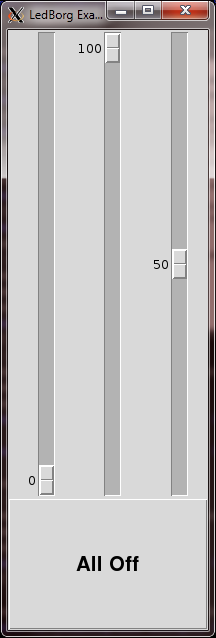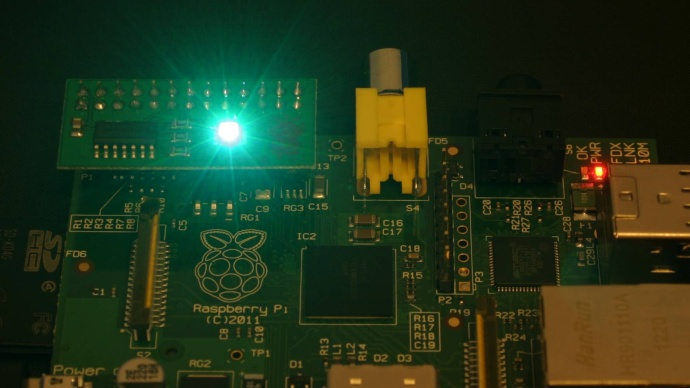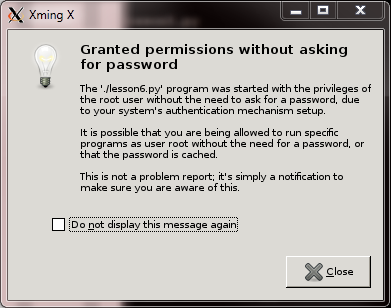# Lesson plan

Installation of the new LedBorg software
Lesson 1. Our first Python example
Lesson 2. Simplifying the code
Lesson 3. Produce a sequence of colours
Lesson 4. Taking user input
Lesson 5. Brightness levels (PWM)
Lesson 6. A simple dialog (GUI)
Lesson 7. Using a pre-made dialog

## A simple dialog (GUI)

If we want to experiment with the various possible LedBorg colour combinations, it would be great if we could run a dialog (GUI) which allows use to control the red, green, and blue channels directly.We can modify the lesson5.py script to use this new method, start by copying the old script:
`cp lesson5.py lesson6.py`
Then open up the new script in your editor and remove all the lines from line 30 onwards.
We will be using the Tkinter library to do most of the heavy lifting for us, dialogs can be a lot of code to write!
To use the library we need to import it first, add the line highlighted below:
```#!/usr/bin/env python

# Import library functions we need
import time
import Tkinter
import wiringpi2 as wiringpi
wiringpi.wiringPiSetup()
```

### Constructing a dialog

For us to make a dialog using Tkinter we need to make a class to represent our dialog.
A class is like a template or blueprint for constructing an object, it defines functions which tell the system how that object will work.
We will write one of these classes, then create an object from it which will be our dialog shown on the screen.
Let us start writing the class:
```# Class representing the GUI dialog
class LedBorg_tk(Tkinter.Tk):
# Constructor (called when the object is first created)
def __init__(self, parent):
# Setup the parent object
Tkinter.Tk.__init__(self, parent)
self.parent = parent
self.protocol("WM_DELETE_WINDOW", self.OnExit) # Call the OnExit function when user closes the dialog
```
This first part of the class has a lot of parts to it, most of which will be common for any dialog class we would write.

Line 32 tells Python we are making a new class called `LedBorg_tk`, which will inherit from the class `Tkinter.Tk`
When a class inherits another it gains all of the functionality of that class, in our case that class deals with all the nasty code which works out where the mouse is, how to resize the dialog, if the user just clicked on something and so on.
We use inheritance like this so that we do not have to write everything again each time we need to create a new dialog, it saves a massive amount of typing!

Line 34 defines a function called `__init__`, this is a special function because it gets called when a new object is created using this class.
The self variable is the object itself, which all functions belonging to a class will have as their first value.
The parent variable is whichever container this dialog lives in (part of how Tkinter works), we will come back to that later on but it is not normally important for dialog objects.

Line 36 gets the inherited class, `Tkinter.Tk`, to call its own `__init__` function, in most cases of inheritance this should be the first thing you do inside your `__init__` function.

Line 37 makes a note of who the parent object is in a variable which belongs to this object.
This is also common practice for `__init__` functions.

Finally line 38 tells Tkinter we want a function called `OnExit` to be called when the user closes this dialog.
We will need to create this function before we are done.

We will also make some variables to let us know what colour has been set:
```        # Set any state variables
self.red = 0
self.green = 0
self.blue = 0
```
These variables work the same way as the variables we have used before, but the `self.` at the start of their name means that they belong to the object, in this case our dialog.

### Creating some sliders

Now we have a dialog without anything on it we need to decide how we will control the LedBorg.
One way of controlling the level of something is with a slider:
```        # Setup a grid of 3 sliders which control red, green, and blue
self.grid()
self.sldRed = Tkinter.Scale(self, from_ = LED_MAX, to = 0, orient = Tkinter.VERTICAL, command = self.sldRed_move)
self.sldRed.set(0)
self.sldRed.grid(column = 0, row = 0, rowspan = 1, columnspan = 1, sticky = 'NSEW')
```
Line 44 sets up a grid which we can use to tell each object where it belongs on the screen.
We will come back to how it is sized later on.

Line 45 creates our slider object, which is known as a Scale in Tkinter.
We set the maximum and minimum values the slider can produce to be the same as the PWM range, and make it a vertical slider (they can also be horizontal).
Finally we tell Tkinter to call a function called `sldRed_move` when the slider position gets changed.
We will need to create the function itself later.

Line 46 changes the sliders position to 0, the minimum value.

Line 47 tells the slider to put itself on the first row, first column of the grid, it will take a grid slot of size 1 × 1.

Now we have one slider to control red, we can make another two for green and blue, but this time in the second and third columns:
```        self.sldGreen = Tkinter.Scale(self, from_ = LED_MAX, to = 0, orient = Tkinter.VERTICAL, command = self.sldGreen_move)
self.sldGreen.set(0)
self.sldGreen.grid(column = 1, row = 0, rowspan = 1, columnspan = 1, sticky = 'NSEW')
self.sldBlue = Tkinter.Scale(self, from_ = LED_MAX, to = 0, orient = Tkinter.VERTICAL, command = self.sldBlue_move)
self.sldBlue.set(0)
self.sldBlue.grid(column = 2, row = 0, rowspan = 1, columnspan = 1, sticky = 'NSEW')
```

### Placing a button

Now we have some sliders we will add a button below them.
To make the button look sensible we want it to span all three columns, meaning the .grid line needs a columnspan of 3:
```        # Make an Off button
self.butOff = Tkinter.Button(self, text = 'All Off', command = self.butOff_click)
self.butOff['font'] = ("Arial", 20, "bold")
self.butOff.grid(column = 0, row = 1, rowspan = 1, columnspan = 3, sticky = 'NSEW')
```
The button is slightly simpler to set up compared to the sliders.
We set the text “All off” to be shown on the button, and tell the button which function to call when it is clicked.
Notice we also set a property, the font, by using a separate call after creating the button.
We could have set the font in the same line we set the text, but the nice thing about how we set the font is that it allows us to change settings after the button has been created.
This can be useful if you wanted to change the text, for example after the user tells you their name.

### Setting the grid layout

Now we need to set the shape our grid looks:
```        # Set our grid layout, make the sliders taller than the button
self.grid_columnconfigure(0, weight = 1)
self.grid_columnconfigure(1, weight = 1)
self.grid_columnconfigure(2, weight = 1)
self.grid_rowconfigure(0, weight = 4)
self.grid_rowconfigure(1, weight = 1)
```
The weight property determines how Tkinter scales column and row sizes, the larger the number the quicker a row or column gets larger to fill the dialog.
We make all three columns the same weight which should make them equally sized, we make the first row grow faster than the second row by giving it a larger weight, this means that the sliders should be taller than the button.

### Finishing the initialisation

Once the `__init__` function completes, the dialog will become visible.
We need to set some final options:
```        # Set the size and name of the dialog
self.resizable(True, True)
self.geometry('200x600')
self.title('LedBorg Example GUI')
# Setup the initial colour
SetLedBorg(self.red, self.green, self.blue)
```
This makes the dialog resizable in both width and height, 200 × 600 pixels starting size, gives it a title, and finally finishes by making sure the LedBorg has its initial colour set.

Now we have set up how our dialog looks, we need to tell it how it works.
We do this by setting up the five functions below that we named earlier in the code.

### The OnExit function

We told Tkinter to call a function named `OnExit` when the user tells the dialog to close (clicks the X in the top-right corner).
This function will turn the LedBorg off, then tell the dialog to finish running:
```    # Called when the user closes the dialog
def OnExit(self):
# Turn the LedBorg off and end the program
LedBorgOff()
self.quit()
```

### The slider movement functions

Each slider had a differently named function that would be called when it is moved.
Here is the `sldRed_move` function:
```    # Called when sldRed is moved
def sldRed_move(self, value):
# Update the red value then set the colour again
self.red = float(value) / LED_MAX
SetLedBorg(self.red, self.green, self.blue)
```
Notice this function gets passed a value, in this case the position the slider has been moved to.
First we take that value and convert it to the 0 to 1 range (we use the float function to ensure we will get an answer including a fractional part), then assign it to the appropriate variable.
Secondly we set the LedBorg colour using the three colour variables which belong to the dialog object.

The `sldGreen_move` and `sldBlue_move` functions are nearly identical:
```    # Called when sldGreen is moved
def sldGreen_move(self, value):
# Update the green value then set the colour again
self.green = float(value) / LED_MAX
SetLedBorg(self.red, self.green, self.blue)

# Called when sldBlue is moved
def sldBlue_move(self, value):
# Update the blue value then set the colour again
self.blue = float(value) / LED_MAX
SetLedBorg(self.red, self.green, self.blue)
```

### The butOff_click function

The final function happens when we click the all off button:
```    # Called when butOff is clicked
def butOff_click(self):
self.sldRed.set(0)
self.sldGreen.set(0)
self.sldBlue.set(0)
```
This function moves all three sliders to the off position.
When each slider is moved its movement function will be called as if the user had moved the slider themselves, therefore each call will not only move the slider back to 0, it will also turn that colour off for us as well.

### Running the dialog

We have now completely coded our dialog, all that remains is to get Python to make one:
```# Create a copy of the LedBorg GUI and pass control to it
app = LedBorg_tk(None)
app.mainloop()
```
Line 102 creates a new dialog by using the class definition we created on lines 31 to 99.
Remember the parent variable way back at the start, the None value we pass is our way of telling Python there is no parent in this case.

Our final line, 103, tells Python to pass control to the dialog until it is finished.
This has the effect of holding our program at this line until the self.quit() call back on line 75.

### The complete script

The completed script can be downloaded directly to the Raspberry Pi using:
`wget -O lesson6.py http://www.piborg.org/downloads/ledborg-new/lesson6.txt`
Remember we make the script executable with:
`chmod +x lesson6.py`
this time we need to run it with gksudo instead of sudo:
`gksudo ./lesson6.py`

gksudo is similar to sudo, but it also allows access to drawing things on the screen.
The biggest problem with gksudo is that errors in our script which cause it to end abruptly (like using a variable which does not exist) will cause our script to end without printing the normal error message, this can make fixing errors difficult.
Secondly we may get presented with the following dialog:You can safely ignore this warning.

```#!/usr/bin/env python

# Import library functions we need
import time
import Tkinter
import wiringpi2 as wiringpi
wiringpi.wiringPiSetup()

# Setup software PWMs on the GPIO pins
PIN_RED = 0
PIN_GREEN = 2
PIN_BLUE = 3
LED_MAX = 100
wiringpi.softPwmCreate(PIN_RED,   0, LED_MAX)
wiringpi.softPwmCreate(PIN_GREEN, 0, LED_MAX)
wiringpi.softPwmCreate(PIN_BLUE,  0, LED_MAX)
wiringpi.softPwmWrite(PIN_RED,   0)
wiringpi.softPwmWrite(PIN_GREEN, 0)
wiringpi.softPwmWrite(PIN_BLUE,  0)

# A function to set the LedBorg colours
def SetLedBorg(red, green, blue):
wiringpi.softPwmWrite(PIN_RED,   int(red   * LED_MAX))
wiringpi.softPwmWrite(PIN_GREEN, int(green * LED_MAX))
wiringpi.softPwmWrite(PIN_BLUE,  int(blue  * LED_MAX))

# A function to turn the LedBorg off
def LedBorgOff():
SetLedBorg(0, 0, 0)

# Class representing the GUI dialog
class LedBorg_tk(Tkinter.Tk):
# Constructor (called when the object is first created)
def __init__(self, parent):
# Setup the parent object
Tkinter.Tk.__init__(self, parent)
self.parent = parent
self.protocol("WM_DELETE_WINDOW", self.OnExit) # Call the OnExit function when user closes the dialog
# Set any state variables
self.red = 0
self.green = 0
self.blue = 0
# Setup a grid of 3 sliders which control red, green, and blue
self.grid()
self.sldRed = Tkinter.Scale(self, from_ = LED_MAX, to = 0, orient = Tkinter.VERTICAL, command = self.sldRed_move)
self.sldRed.set(0)
self.sldRed.grid(column = 0, row = 0, rowspan = 1, columnspan = 1, sticky = 'NSEW')
self.sldGreen = Tkinter.Scale(self, from_ = LED_MAX, to = 0, orient = Tkinter.VERTICAL, command = self.sldGreen_move)
self.sldGreen.set(0)
self.sldGreen.grid(column = 1, row = 0, rowspan = 1, columnspan = 1, sticky = 'NSEW')
self.sldBlue = Tkinter.Scale(self, from_ = LED_MAX, to = 0, orient = Tkinter.VERTICAL, command = self.sldBlue_move)
self.sldBlue.set(0)
self.sldBlue.grid(column = 2, row = 0, rowspan = 1, columnspan = 1, sticky = 'NSEW')
# Make an Off button
self.butOff = Tkinter.Button(self, text = 'All Off', command = self.butOff_click)
self.butOff['font'] = ("Arial", 20, "bold")
self.butOff.grid(column = 0, row = 1, rowspan = 1, columnspan = 3, sticky = 'NSEW')
# Set our grid layout, make the sliders taller than the button
self.grid_columnconfigure(0, weight = 1)
self.grid_columnconfigure(1, weight = 1)
self.grid_columnconfigure(2, weight = 1)
self.grid_rowconfigure(0, weight = 4)
self.grid_rowconfigure(1, weight = 1)
# Set the size and name of the dialog
self.resizable(True, True)
self.geometry('200x600')
self.title('LedBorg Example GUI')
# Setup the initial colour
SetLedBorg(self.red, self.green, self.blue)

# Called when the user closes the dialog
def OnExit(self):
# Turn the LedBorg off and end the program
LedBorgOff()
self.quit()

# Called when sldRed is moved
def sldRed_move(self, value):
# Update the red value then set the colour again
self.red = float(value) / LED_MAX
SetLedBorg(self.red, self.green, self.blue)

# Called when sldGreen is moved
def sldGreen_move(self, value):
# Update the green value then set the colour again
self.green = float(value) / LED_MAX
SetLedBorg(self.red, self.green, self.blue)

# Called when sldBlue is moved
def sldBlue_move(self, value):
# Update the blue value then set the colour again
self.blue = float(value) / LED_MAX
SetLedBorg(self.red, self.green, self.blue)

# Called when butOff is clicked
def butOff_click(self):
self.sldRed.set(0)
self.sldGreen.set(0)
self.sldBlue.set(0)

# Create a copy of the LedBorg GUI and pass control to it
app = LedBorg_tk(None)
app.mainloop()
```

Continue to lesson 7. Using a pre-made dialog